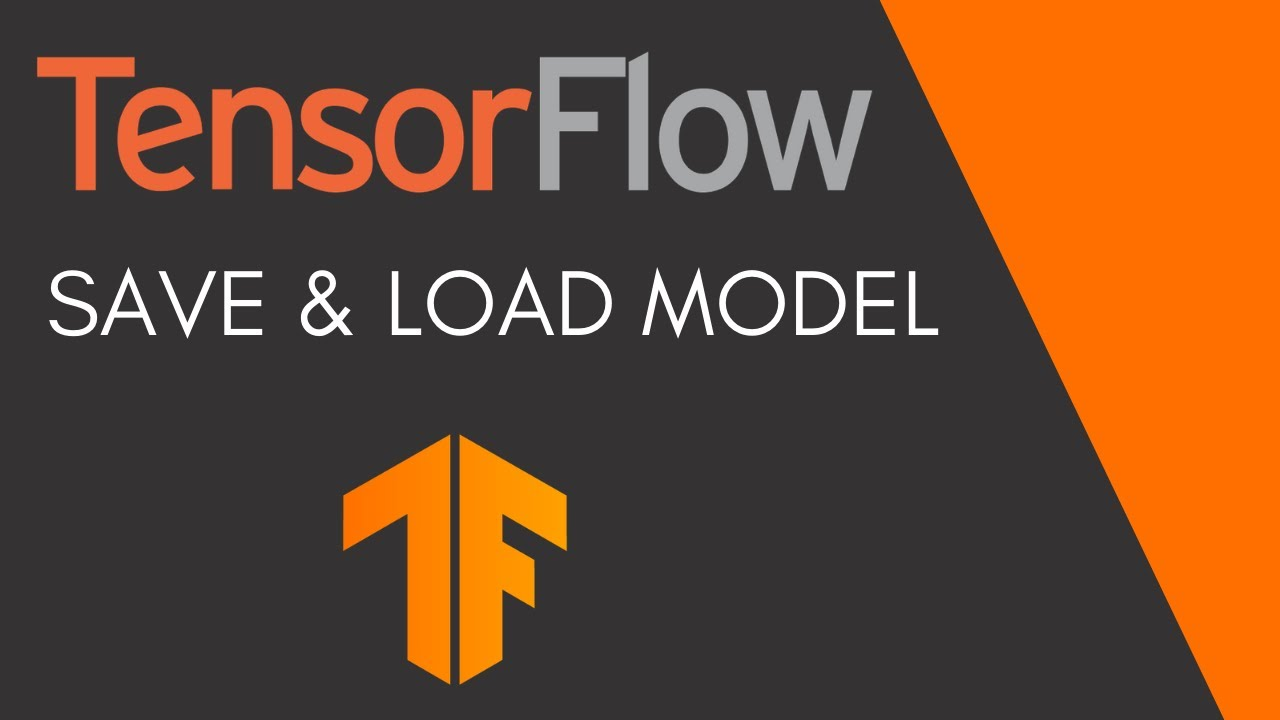In this video I'm going to show you how to save and load model weights and then also how you can save and load the entire model including the architecture and optimizer states. The saved model will also be able to run on other TensorFlow frameworks such as TensorFlow.js and TensorFlow Lite without any model conversions.

In this video I'm going to show you how to save and load model weights and then also how you can save and load the entire model including the architecture and optimizer states. The saved model will also be able to run on other TensorFlow frameworks such as TensorFlow.js and TensorFlow Lite without any model conversions. This process of saving the entire model (to a data structure that can then be run on any of these frameworks) is referred to as serialization.

## What is TensorFlow? TensorFlow

An end-to-end open-source platform for Machine Learning. Before we start with TensorFlow, we will need to know what machine learning and deep learning technologies are.

## A TensorFlow Modeling Pipeline using TensorFlow Datasets and TensorBoard

This article investigates TensorFlow components for building a toolset to make modeling evaluation more efficient. Specifically, TensorFlow Datasets (TFDS) and TensorBoard (TB) can be quite helpful in this task.

## Keras vs. Tensorflow - Difference Between Tensorflow and Keras

Keras vs Tensorflow - Learn the differences between Keras and Tensorflow on basis of Ease to use, Fast development,Functionality,flexibility,Performance etc

## Deployment of a TensorFlow model to Production using TensorFlow Serving

Deploy a Deep Learning Model to Production using TensorFlow Serving.

## A TensorFlow Modeling using TensorFlow Datasets and TensorBoard

While completing a highly informative AICamp online class taught by Tyler Elliot Bettilyon (TEB) called Deep Learning for Developers, I …